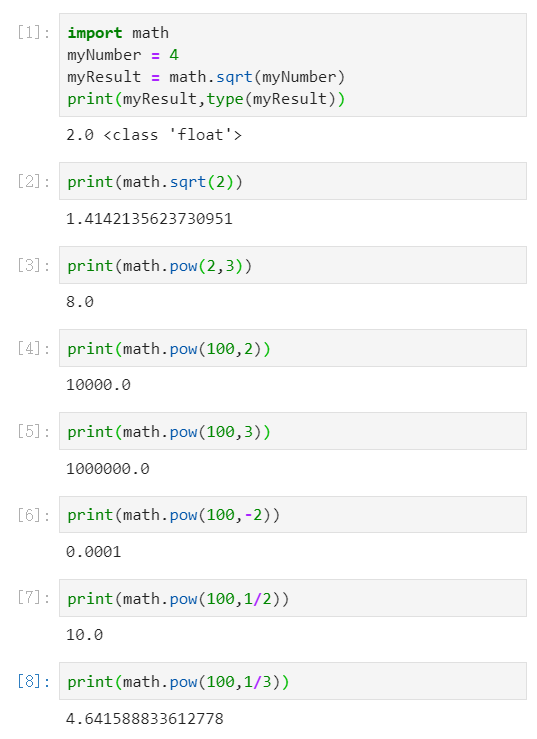### 【Python乘方运算和开平方运算】

【小白从小学Python、C、Java】
【Python-计算机等级考试二级】
Python乘方运算和开平方运算
math.pow()、math.sqrt()方法

import math
myNumber = 4
myResult = math.sqrt(myNumber)
print(myResult,type(myResult))
A 2.0 <class ‘int’>
B 2.0 <class ‘float’>
C 2 <class ‘int’>
D 2 <class ‘float’>

1.math.pow(x,y)方法以浮点数类型返回x的y次方，包括对x进行平方、立方、开方等计算（如下图），并最少保留一位小数。
2.math.sqrt(Number)方法以浮点数类型返回myNumber的平方根，并最少保留一位小数。
2.pow()、sqrt()是不能直接访问的，需要导入 math 模块，通过math.pow()、math.sqrt()调用该方法。
3.题目中myNumber=4，算得平方根为2，由于math.sqrt()方法返回浮点数结果，且最少保留一位小数，则myResult=2.0，数据类型为float，答案为B。